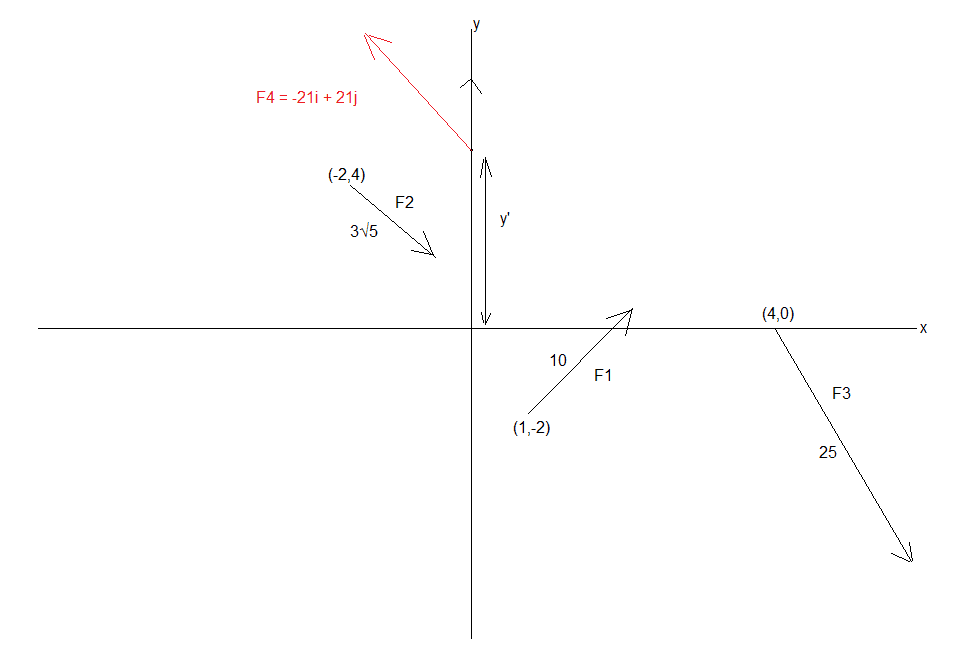# To find the equivalent force

Homework Statement:
Find the equivalent force
Relevant Equations:
Balance of moments

Three forces of magnitudes 10, 3 * sqrt(5) and 25 newtons act along lines whose vector equations are respectively:

r1 = i - 2j + k(4i + 3j) , r2 = -2i + 4j + k(2i - j) and r3 = 4i + k(7i - 24j)

A fourth force F4 is introduced which reduces the system to equilibrium.

Find F4 and the vector equation of its line of action:

I get the same answer as the book for the first part, F4 = -21i + 21j but not for the second part.

I take moments about -2i + 4j to find the y-intercept of the line of action and I am led to the equation r=-(8/21)i+k(-i+j) but the book answer is r=(22/21)i + k(-i+j).

Can anyone help confirm if it is the book or I (as I suspect) that is worng?

Thanks,
Mitch.

haruspex
Homework Helper
Gold Member
2020 Award
I take moments about -2i + 4j to find the y-intercept of the line of action and I am led to the equation r=-(8/21)i+k(-i+j) but the book answer is r=(22/21)i + k(-i+j).
I get 92/21.We know from the first part that:

F1 = 8i + 6j
F2 = 6i-3j
F3 = 7i-24j

Resolving horizontally and vertically gives us F4 = -21i+21j

Taking anti-clockwise moments about (-2,4) gives:

8*6 + 6*3 + 7*4 - 21 * 6 = 21 * (y' - 4) + 21 * 2

This leads to y' = -8/21 and so to an equation of y = -x - 8/21 and so when y = 0 then x = -8/21 hence my answer of r=-(8/21)i+k(-i+j)

Thanks,
Mitch.

haruspex
Homework Helper
Gold Member
2020 Award
8*6 + 6*3 + 7*4 - 21 * 6 = 21 * (y' - 4) + 21 * 2
THere's a typo, but probably just in making the post. I think you meant
8*6 + 6*3 + 7*4 - 24 * 6 = 21 * (y' - 4) + 21 * 2
But shouldn’t it be
8*6 + 6*3 + 7*4 - 24 * 6 + 21 * (y' - 4) + 21 * 2 = 0
?

Thanks so much (yes it was a typo in posting). I misread the question as saying that the forces were replaced by F4 which was to be equilvalent to the initial forces, but of course that's not what it is asking, it is saying that F4 is added in to the system to create equilibrium.

Thanks again,
Mitch.

Thanks so much (yes it was a typo in posting). I misread the question as saying that the forces were replaced by F4 which was to be equilvalent to the initial forces, but of course that's not what it is asking, it is saying that F4 is added in to the system to create equilibrium.

Thanks again,
Mitch.
And I misread the book answer as 22/21 when it is 92/21

haruspex